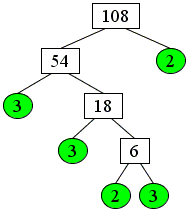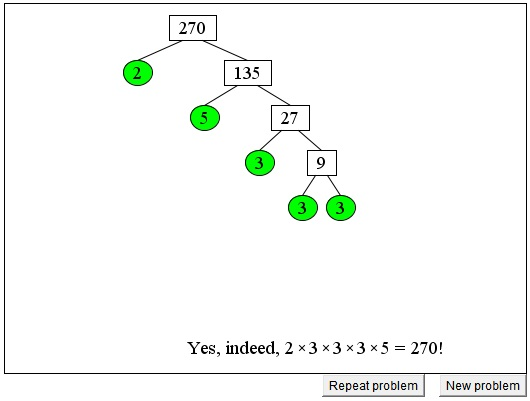Factoring with the Factor Tree

Factoring, i.e., listing all the prime factors, of an integer is a useful skill that often helps to solve math problems. For example, one of the ways to find the GCD (Greatest Common Divisor) or LCM (Least Common Multiple) of two integers is by listing all their prime factors. The GCD is then the product of all the common factors; the LCM is the product of all the remaining ones.

The applet below is a tool for finding such prime factorizations with the device known as the Factor Tree, meaning that you proceed a step at a time. At the beginning you are given a number, say N, which is to be factored and two boxes beneath it. The boxes are to be filled with two numbers, say, N1 and N2 - factors of N - such that N = N1 × N2. (You only need to find one factor. If one is found correctly, the other is filled out automatically. (In "real life," you may use a calculator or the long division algorithm.) Note that the two numbers need not be prime. The one which is not will grow two boxes beneath it so that you'll be able to proceed with further factoring until all the prime factors have been found.

Do not be intimidated if you can't list all the factors right away. You do not have to. Just finding one factor will simplify the problem. Remember the divisibility rules. An even number is divisible by 2. A number is divisible by 3 if the sum of its digits is divisible by 3. The number that ends in 5 is divisible by 5 and the one that ends in 10 is divisible by 10.

While the prime factorization is unique (up to the order of factors), the factor tree reflects the order in which the factors were found, and is by no means unique. So you do not have to worry about finding a "correct" factor at any step. Any factor will do. Check the three examples of factoring of 108:(There is a button at the bottom of the applet that allows you to repeat a problem and see for yourself that different factor trees still produce the same prime factorizaion for any number.)

At any time there may be several boxes to type in the factors. Choose any of them. After typing in a number Press the Enter key. How do you choose a box? You may click on it or you may press the Tab key 1 or more times to get to the box of your choice.

If you are reading this, your browser is not set to run Java applets. Try IE11 or Safari and declare the site https:///www.cut-the-knot.org as trusted in the Java setup.What if applet does not run?

(Elsewhere we use two factor trees simultaneously to find the greatest common divisor and the least common multiple of two numbers.)• GCD and LCM via Factor Tree
• GCD and LCM by Plain Factorization
• Common Multiples and the Least Common Multiple
• GCD(M, N) x LCM(M, N) = M x N
• Divisibility Criteria
• Euclid's Algorithm
• Algorithm for Computing the LCM
• Factors And Multiples
• Two Properties of Greatest Common Divisor
• Properties of GCD and LCM
• A Line in a Square Grid
• Number of Factors of an Integer
• Extension of Euclid's Game
•